# How To Use ISIS Program Within Proteus Assignment Answer

Now place a sine wave generator at the bridge input. Select Main mode - component and P (as before). Choose the library “asimmdls” and select VSINE. Place VSINE onto the schematic (fig 5).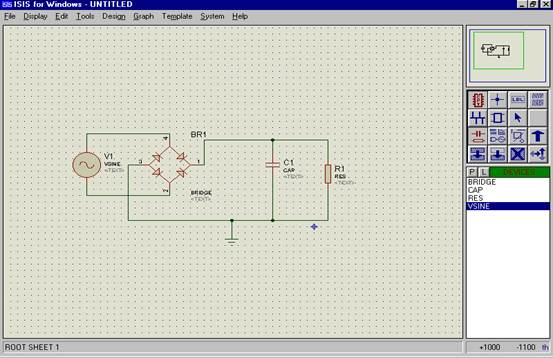Fig 5

Step 7

The following design parameters are to be used:

20V RMS supply (Peak of 20 * v2 = 28.28V) at 50 Hz.

Peak to peak ripple of no more than 1.5V

From the above we must calculate the capacitor value.

Recall from lecture notes that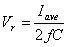where(assume two diode drops gives 1.4 V)

Iave = 26.13 mA

C = 174.23 µF

## Step 8

The schematic components must be edited to give them the required values.

For example, right-click the resistor to highlight it then left click to bring up the edit box. Change RES to 1k (fig 6).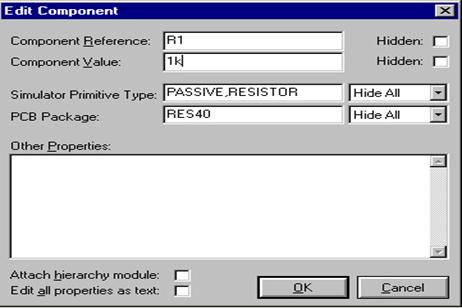Fig 6

Continue to edit all other components. Note that:

k = kilo

u = micro

M = mega

p = pico

n = nano

## Step 9

Similarly right click VSINE and then left click to open the edit box and enter the design parameters as in fig 7: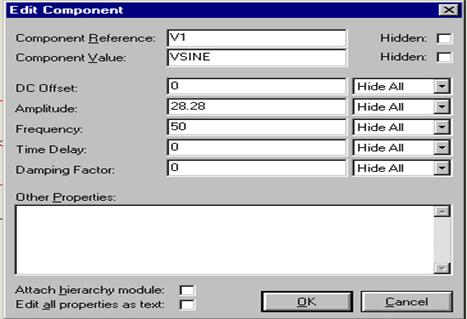Fig 7

## Step 10

To begin simulation we must first select “Gadget Mode” and then “Graph” (fig 8).

Then select “Analogue” and draw a rectangular graph somewhere on the schematic.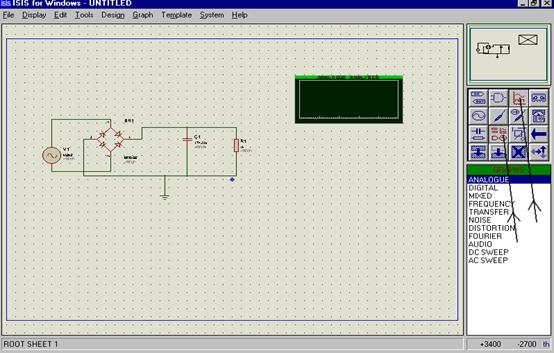Fig 8

Assignment Help | Programming Assignment Help | Online Tutoring | Sample Homework